Articles on LZC, HVAC, PC & CAD too!

## A quick overview of thermal transmittance (U), thermal conductivity (K or λ) & thermal resistance (R)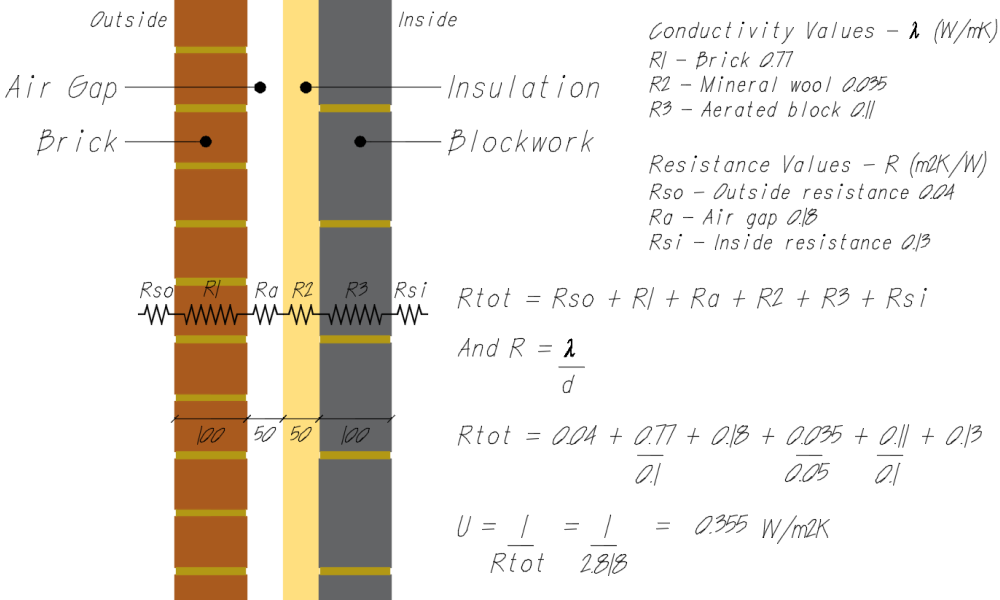U-values indicate the rate of heat transfer (thermal transmittance) through a material or structure and are used to calculate building heat losses (see heat loss calculations).

The U-value is a measure of the heat flow per square metre divided by the temperature difference between inside and outside.

A low U-value is required to save energy. This is achieved when a material has thermal properties providing low conductivity (see thermal conductivity) and high resistance (see thermal resistance).

### What is a U-value?

U-values indicate the rate of heat transfer (thermal transmittance) through a material or structure. U-values are used to calculate building fabric heat losses - the heat transfer through the building fabric itself, i.e. the walls, roof, floor and windows.

The U-value (with units W/m2K) is the rate of heat transfer (through a structure), divided by the difference in temperature across that structure (inside and the outside of a building). The lower the U-value figure, the slower the rate of heat loss, and hence reduced demand on heating or cooling - high insulation levels can contribute to overheating in summertime, but this is another subject.

The rate of heat loss will vary according to the thermal properties of a material. The thermal resistances of the components of a building element are added in series to give a total thermal resistance (R-value - m2K/W). The U-value of the building element is found from the reciprocal of the total thermal resistance.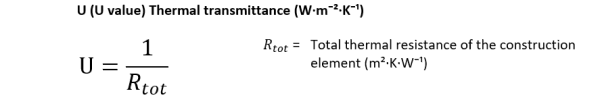A low U-value is required to save energy. This is achieved when a material has thermal properties providing low conductivity (see thermal conductivity) and high resistance (see thermal resistance).

### Thermal Conductivity

Thermal conductivity (k or lambda (λ) value - W/mK) is the capacity of a material to conduct heat assuming there is a temperature gradient across the material.

Thermal conductivity is the time rate of steady-state heat flow through a unit area of a homogeneous material induced by a unit temperature gradient in a direction perpendicular to that unit area. Thermal conductivity is a constant for a particular material. It is a fundamental material property independent of thickness.

The thermal conductivity of a typical mineral fibre product is in the range of 0.034 to 0.044 W/mK, whereas a good rigid phenolic insulation thermal conductivity is in the range of 0.021 to 0.024 W/mK. Therefore for the same thickness phenolic is a better insulator than mineral fibre. Find out more about thermal insulation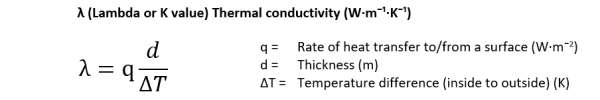Thermal conductivity data for several common building materials for design purposes are given in BS EN ISO 10456 (2007). Also see CIBSE Guide A (2015) Appendix 3.A7 which provides properties for an extensive range of materials and Appendix 3.A8 for thermal properties for typical constructions.

### Thermal Resistance

Thermal resistance (R-value - m2K/W) is the capacity of a product to resist against heat loss. A product with a higher R-value will perform better as an insulator.

The thermal resistance of a specific product can be found by dividing its thickness by the thermal conductivity or if the R-value is given, then the thermal conductivity can be calculated by dividing the material thickness by the R-value.

Where the thermal conductivity is known, the thermal resistance is found from the following equation:The thermal resistances of the components of a building element, such as a cavity wall, can be added in series to give a total thermal resistance. The sum of the thermal resistance is then used to calulate the U-value (see U-value calculation).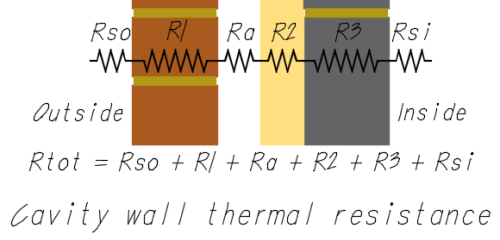### Heat Loss Calculations

Having established the construction U-values, the fabric (conduction) heat losses (W) can be found. The heat loss for a surface can be estimated from the following:

Q = U × A × (Ti-To)

Where:

• Q = heat loss (W)
• U = the ‘U-value’ —the overall thermal conductivity or transmittance (W/m2.K)
• A = the surface area of the particular fabric (m2)
• Ti = the inside temperature (K or °C)
• To = the outside temperature (K or °C)

It can be seen that for a given area at fixed temperatures, a lower U-value will return a lower heat loss.

### Thermal Insulation

Energy usage in buildings is strongly related to the fabric insulation properties. Heat flows from inside to outside surfaces of the building fabric by the process of conduction. Thermal insulation with good thermal properties (low conductivity and high resistance) reduces this heat flow to a minimum.

The required thickness T (in millimetres) can be estimated from the target U-Value (U in W/(m2K)) and the respective material's thermal conductivity K (in W/mK) by using the following formula: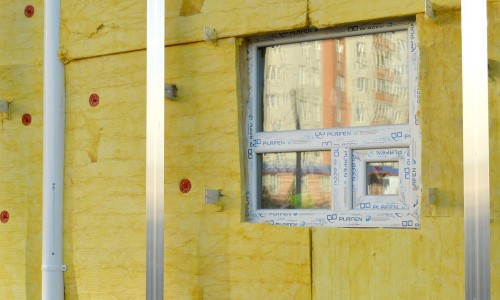Refer to CIBSE Guide A (2015) section 3 Thermal properties of building structures and Appendix 3.A7 which provides properties for an extensive range of materials and Appendix 3.A8 for thermal properties for typical constructions.

Also see Building Research Establishment's BR 443 and BS EN ISO 6946:2017.

2009-2022 All Rights Reserved - www.homemicro.co.uk    Based upon an original design by w3.css with a little help from Bootstrap & Pixaby / free images